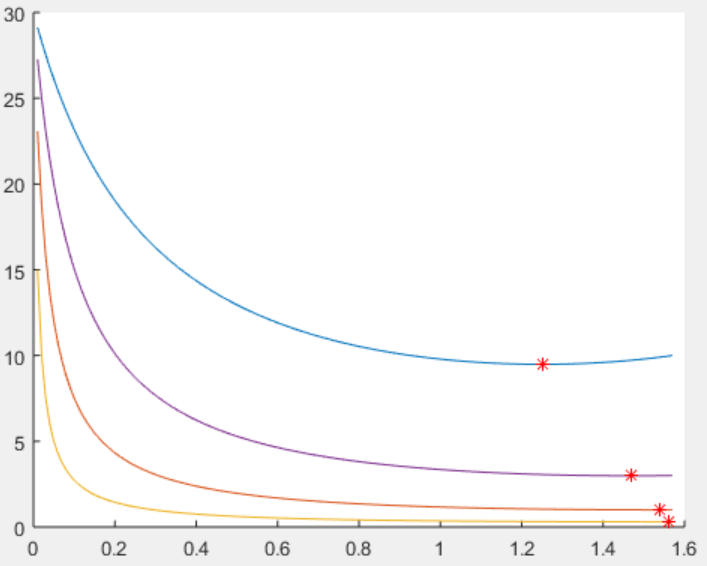## Introduction

What is the best angle at which to cross the road? Sidewalks run perpendicular to the road, but might it not be safer in some cases to cross at an angle closer to the car's direction of travel?

Here we consider crossing a one-way road with one vehicle approaching. Then crossing at an angle other than perpendicular increases the walking time, and doing so makes sense as long as this increase in time, multiplied by the vehicle's speed, results in a shorter distance than the additional distance gained along the road. But this isn't really true, because the vehicle has to never intersect the crossing path. To consider this situation a short matlab script is written.

## Results

The plot below shows the required crossing speed at different conditions. The red * marks the minimum safe crossing speed. The y-axis is in m/s so this might be called running speed. The x-axis is the angle of the crossing path with the road in radians, where 0 is running along with the car and pi/2 (1.57) is directly perpendicular to the road. The traces increase in y as the vehicle speed is raised and as its distance to the crossing is decreased. Thus the lowest trace, indicating minimum crossing speed at almost pi/2, is true when the vehicle is slow or far away. As the vehicle gets closer or faster, the safe crossing angle decreases to about 1.48 or 5 degrees off perpendicular, going a bit along the car's travel direction. But at the same time, the minimum crossing speed rises significantly, quickly overcoming human ability. This means that for a practical scenario, it is always better to run immediately towards the curb than to attempt calculating an optimal angle!Overall, optimal theta is affected by the ratio da/dc, where large da/dc=perpendicular travel, small da/dc=parallel travel. This means it is much preferred to run towards side closest to curb (because of proportional relationship the factor change in da/dc may be significant) and if the car is really close run slightly forward. However for the considered scenario of a 60mph car at about 100ft the along-car distance that the optimal angle provides is 1 inch, so it will not pay off to think about the optimal angle in that situation - just run towards the curb as directly as possible. On the other hand if the car is really close (10 ft) running away from it as well as towards the curb gives an advantage of 11 inches and also gives the driver an extra 0.0055 seconds to come to a safe speed or swerve out of the way - which will hopefully be the case because it will not be possible to run out of the way of a car at a constant 60 mph if it is that close (it would require running at 9.5 m/s = 21.25 mph = 2 min 50 sec per mile!).